## RD Sharma Class 8 Solutions Chapter 11 Time and Work Ex 11.1

These Solutions are part of RD Sharma Class 8 Solutions. Here we have given RD Sharma Class 8 Solutions Chapter 11 Time and Work Ex 11.1

Question 1.
Rakesh can do a piece of work in 20 days. How much work can he do in 4 days ?
Solution:
Rakesh can do it in 20 days = 1
his 1 day’s work = $$\frac { 1 }{ 20 }$$
and his 4 days work = $$\frac { 1 }{ 20 }$$ x 4 = $$\frac { 1 }{ 5 }$$ th work

Question 2.
Rohan can paint $$\frac { 1 }{ 3 }$$ of a painting in 6 days. How many days will he take to complete the painting ?
Solution:
Rohan can paint $$\frac { 1 }{ 3 }$$ of painting in = 6 days
he will complete the painting in = $$\frac { 6 x 3 }{ 1 }$$ = 18 days

Question 3.
Anil can do a piece of work in 5 days and Ankur in 4 days. How long will they take to do the same work, if they work together ?
Solution:
Anil’s 1 day’s work = $$\frac { 1 }{ 5 }$$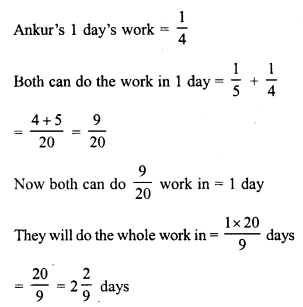Question 4.
Mohan takes 9 hours to mow a large lawn. He and Sohan together can mow it in 4 hours. How long will Sohan take to mow the lawn if he works alone ?
Solution:Question 5.
Sita can finish typing a 100 page document in 9 hours, Mita in 6 hours and Rita in 12 hours. How long will they take to type a 100 page document if they work together?
Solution:
Sita can do a work in 1 hour = $$\frac { 1 }{ 9 }$$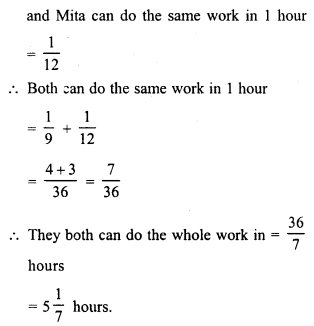Question 6.
A, B and C working together can do a piece of work in 8 hours. A alone can do it in 20 hours and B alone can do it in 24 hours. In how many hours will C alone do the same work ?
Solution: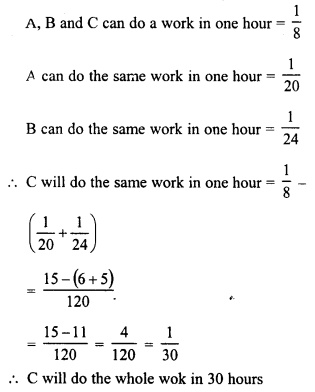Question 7.
A and B can do a piece of work in 18 days; B and C in 24 days and A and C in 36 days. In what time can they do it, all working together ?
Solution:Question 8.
A and B can do a piece of work in 12 days; B and C in 15 days; C and A in 20 days. How much time will A alone take to finish the work ?
Solution: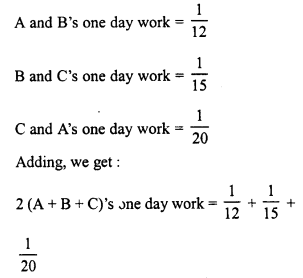Question 9.
A, B and C can reap a field in 15$$\frac { 3 }{ 4 }$$ days; B, C and D in 14 days; C, D and A in 18 days; D, A and B in 21 days. In what time can A, B, C and D together reap it ?
Solution:Question 10.
A and B can polish the floors of a building in 10 days A alone can do $$\frac { 1 }{ 4 }$$ th of it in 12 days. In how many days can B alone polish the floor ?
Solution: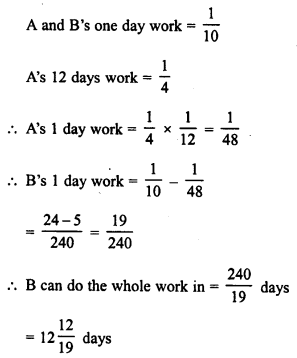Question 11.
A and B can finish a work in 20 days. A alone can do $$\frac { 1 }{ 5 }$$ th of the work in 12 days. In how many days can B alone do it ?
Solution: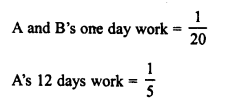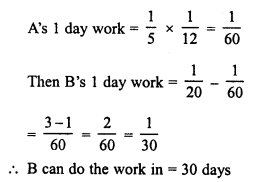Question 12.
A and B can do a piece of work in 20 days and B in 15 days. They work together for 2 days and then A goes away. In how many days will B finish the remaining work ?
Solution: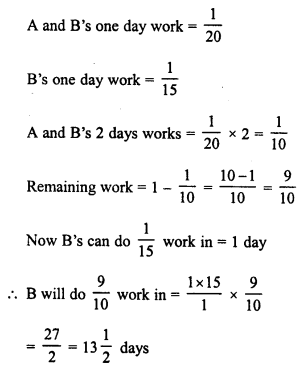Question 13.
A can do a piece of work in 40 days and B in 45 days. They work together for 10 days and then B goes away. In how many days will A finish the remaining work ?
Solution: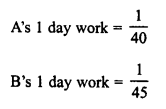Question 14.
Aasheesh can paint his doll in 20 minutes and his sister Chinki can do so in 25 minutes. They paint the doll together for five minutes. At this juncture they have a quarrel and Chinki withdraws from painting. In how many minutes will Aasheesh finish the painting of the remaining doll ?
Solution: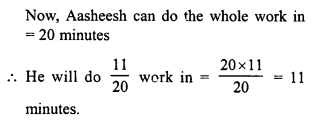Question 15.
A and B can do a piece of work in 6 days and 4 days respectively. A started the work; worked at it for 2 days and then was joined by B. Find the total time taken to complete the work.
Solution: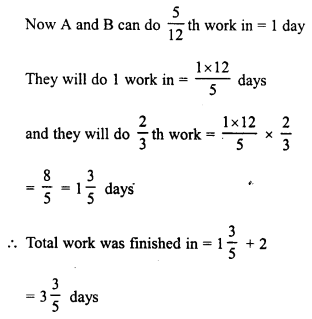Question 16.
6 men can complete the electric fitting in a building in 7 days. How many days will it take if 21 men do the job ?
Solution:
6 men can complete the work in = 7 days
1 man will complete the same work in = 7 x 6 days (Less men, more days)
21 men will finish the work in = $$\frac { 7 x 6 }{ 21 }$$ days (More men, less days) = 2 days

Question 17.
8 men can do a piece of work in 9 days. In how many days will 6 men do it ?
Solution:
8 men can do a work in = 9 days
1 men will do the work in = 9 x 8 days (Less men, more days)
6 men will do the work in = $$\frac { 9 x 8 }{ 6 }$$ days (More men, less days)
= $$\frac { 72 }{ 6 }$$ = 12 days

Question 18.
Reema weaves 35 baskets in 25 days. In how many days will she weave 55 baskets?
Solution:
Reema can weave 35 baskets in = 25 daysQuestion 19.
Neha types 75 pages in 14 hours. How many pages will she type in 20 hours ?
Solution:
Neha types pages in 14 hours = 75 pages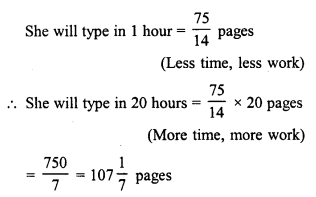Question 20.
If 12 boys earn Rs. 840 in 7 days, what will 15 boys earn in 6 days ?
Solution:
12 boys in 7 days earn an amount of = Rs. 840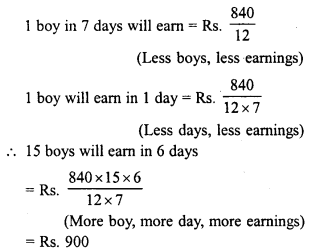Question 21.
If 25 men earn Rs. 1000 in 10 days, how much will 15 men earn in 15 days ?
Solution:
25 men can earn in 10 days = Rs. 1000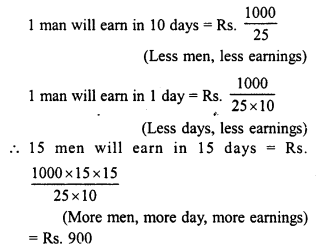Question 22.
Working 8 hours a day, Ashu can copy a book in 18 days. How many hours a day should he work so as to finish the work in 12 days ?
Solution:
Ashu can copy a book in 18 days working in a day = 8 hours
He will copy the book in 1 day working = 8 x 18 hours a day (Less days, more hours a day)
He will copy the book in 12 days working in a day = $$\frac { 8 x 18 }{ 12 }$$ hours
(More days, less hours a day)
= $$\frac { 144 }{ 12 }$$ = 12 hours a day

Question 23.
If 9 girls can prepare 135 garlands in 3 hours, how many girls are needed to prepare 270 garlands in 1 hour.
Solution:
135 garlands in 3 hours are prepared by = 9 girls
1 garland in 3 hours will be prepared by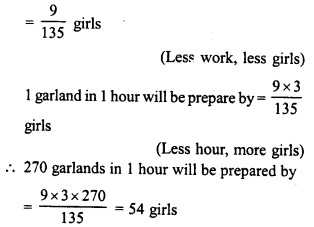Question 24.
A cistern can be filled by one tap in 8 hours, and by another in 4 hours. How long will it take to fill the cistern if both taps are opened together ?
Solution:
First tap’s 1 hour work to fill the cistern = $$\frac { 1 }{ 8 }$$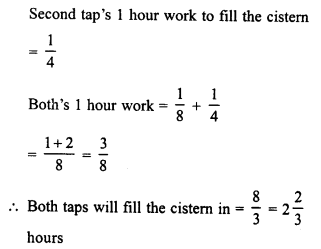Question 25.
Two taps A and B can fill an overhead tank in 10 hours and 15 hours respectively. Both the taps are opened for 4 hours and then B is turned off. How much time will A take to fill the remaining tank ?
Solution: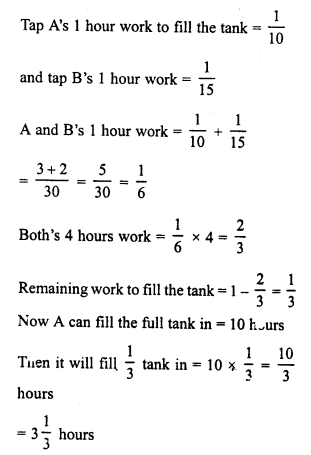Question 26.
A pipe can fill a cistern in 10 hours. Due to a leak in the bottom, it is filled in 12 hours. When the cistern is full, in how much time will it be emptied by the leak?
Solution: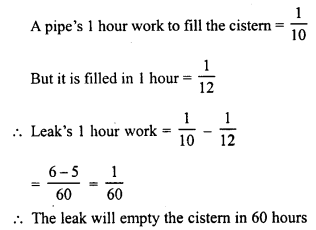Question 27.
A cistern has two inlets A and B which can fill it in 12 hours and 15 hours respectively. An outlet can empty the full cistern in 10 hours. If all the three pipes are opened together in the empty cistern, how much time will they take to fill the cistern completely ?
Solution: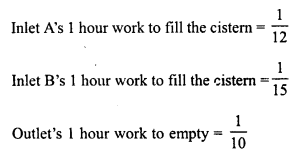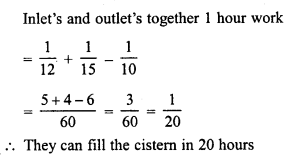Question 28.
A cistern can be filled by a tap in 4 hours and emptied by an outlet pipe in 6 hours. How long will it take to fill the cistern of both the tap and the pipe are opened together ?
Solution: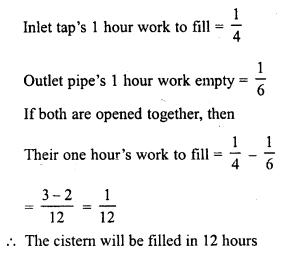Hope given RD Sharma Class 8 Solutions Chapter 11 Time and Work Ex 11.1 are helpful to complete your math homework.

If you have any doubts, please comment below. Learn Insta try to provide online math tutoring for you.